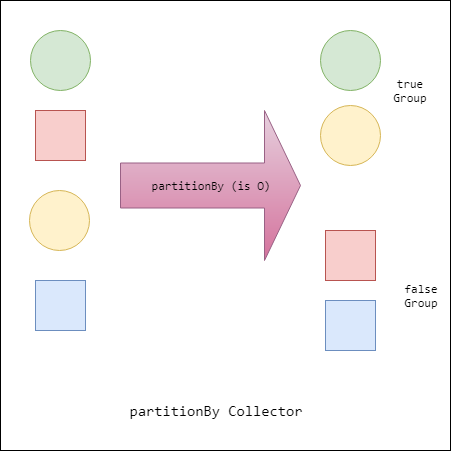## How to Partition data in Stream | Java 8 ?

One important operation that we often perform on `Collection` is partition it into two collections of values. For example, if you’ve got a Stream of `Student`, then you might wish to divide them based on gender. One approach to doing this is to perform two different filters, one looking for female students and the other for male students. This approach has a couple of downsides, though. First, you’ll need two streams in order to perform these two stream operations. Second, if you’ve got a long sequence of operations leading up to your filters, these will need to be performed twice over each stream. This also doesn’t result in clean code. Consequently, there is a collector, `partitioningBy`, that takes a stream and partitions its contents into two groups (see the following figure). It uses a `Predicate` to determine whether an element should be part of the `true group` or the `false group` and returns a `Map from Boolean to a List of values`. So, the Predicate returns `true` for all the values in the true `List` and `false` for the other `List`.We can use the above feature to split out students based on gender. In this case, our partitioning function tells us whether the Student is male or female. Following example is doing the partition by the `partitioningBy `predicate in normal way and using method reference.

```package com.tuturself;

import java.util.ArrayList;
import java.util.List;
import java.util.Map;
import java.util.stream.Collectors;
import java.util.stream.Stream;

public class PartitionTest {

public static void main(String[] args) {
List < Student > students = new ArrayList < > ();

Map < Boolean, List < Student >> maleAndFemale = partition(students.stream());
maleAndFemale.forEach((k, v) -> {
System.out.println(v);
});

// Now partition by method reference
maleAndFemale = partitionByRef(students.stream());
maleAndFemale.forEach((k, v) -> {
System.out.println(v);
});
}

public static Map < Boolean, List < Student >> partition(Stream < Student > students) {
return students.collect(Collectors.partitioningBy(student -> student.isFemale()));
}

// Partitioning up a stream of Students based on gender by method reference
public static Map < Boolean, List < Student >> partitionByRef(Stream < Student > students) {
return students.collect(Collectors.partitioningBy(Student::isFemale));
}
}

class Student {

private int id;
private String name;
private String sex;

public Student(int id, String name, String sex) {
this.id = id;
this.name = name;
this.sex = sex;
}

public boolean isFemale() {
return this.sex.equals("F") ? true : false;
}

@Override
public String toString() {
return "Student [id=" + id + ", " + "name=" + name + ", sex=" + sex + "]";
}
}```

Now output of the program is :

```[Student [id=1, name=Ninja Panda, sex=M], Student [id=2, name=Master Shifu, sex=M]]
[Student [id=3, name=Tigrees, sex=F], Student [id=4, name=Wonder Woman, sex=F]]
[Student [id=1, name=Ninja Panda, sex=M], Student [id=2, name=Master Shifu, sex=M]]
[Student [id=3, name=Tigrees, sex=F], Student [id=4, name=Wonder Woman, sex=F]]
```

CORE JAVA JAVA-8 STREAM